# Catch up on the latest AI articles# Should Cross-entropy Be Used In Classification Tasks?

3 main points
✔️ Compare cross-entropy loss and mean squared error in classification tasks
✔️ Validated on a variety of tasks, including natural language processing, speech recognition, and computer vision
✔️ Models using squared error perform better overall

written by Like HuiMikhail Belkin
(Submitted on 12 Jun 2020 (v1), last revised 4 Nov 2020 (this version, v3))

Subjects: Machine Learning (cs.LG); Machine Learning (stat.ML)## first of all

A commonly used loss function in classification tasks is the cross-entropy loss (CET) rather than the Mean Squared Error (MSE).

Is this really the right thing to do?

In other words, is cross-entropy loss more effective than MSE in the classification task?

In the paper presented in this article, we compared cross-entropy and squared error in various tasks such as natural language processing, speech recognition, and computer vision. The results show that there are many cases where the models trained using the squared error are rather advantageous, such as the models trained using squared error performed as well or better than the models using cross-entropy, and the variance is small with respect to the randomness of initialization.

## experiment

### data set

To compare cross-entropy and squared error, tasks across three domains were used: natural language processing (NLP), speech recognition (ASR), and computer vision (CV). Each experiment was performed on the following datasets.

NLP

• MRPC
• SST-2
• QNLI
• QQP

ASR

• TIMIT
• WSJ
• Librispeech

CV

• MNIST
• CIFAR-10
• ImageNet

### architecture

The architecture used in the experiments is as follows

NLP

ASR

CV

For each task, the statistics of the data set are as follows

NLP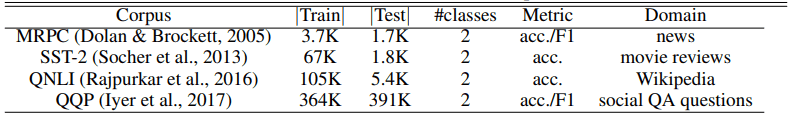ASR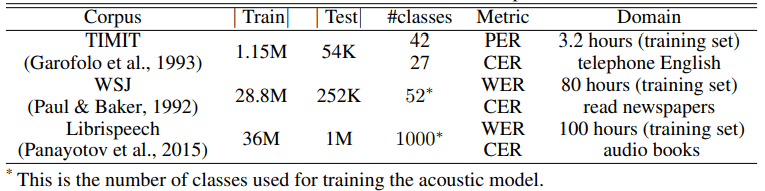CV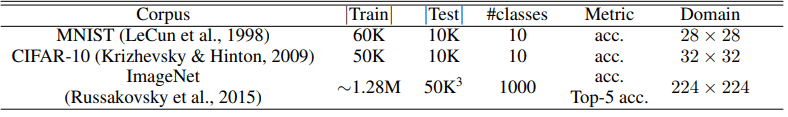### Experimental Protocol

When training with cross-entropy loss, learning was stopped when validation performance did not improve for five consecutive epochs. When training with squared loss, the following two protocols were used.

• Similar to the cross-entropy loss, learning is stopped when validation performance does not improve for 5 consecutive epochs.
• Training with the same number of epochs as the number of epochs when training cross-entropy loss

The latter is designed so that the computational resources are the same for cross-entropy loss and squared loss, which is a favorable setting for cross-entropy loss. In addition, in the experimental results shown below, the average of the experiments with five different random initializations for each task is denoted.

### Important points for implementation

The details of the implementation are given in the original paper, but the most important points are explained below.

#### Deletion of the softmax layer

In the case of cross-entropy loss, there is a softmax layer at the end.

When squared loss is used, this layer is removed.

#### Loss rescaling

For datasets with a large number of output classes (more than 42 in our experiments), we perform loss rescaling to speed up training.

Let $x \in R^d$ be a feature vector, $y \in R^C$ (where C is the number of output classes) be a one-hot vector representing the labels, and the model is represented as $f:R^d→R^C$.

In this case, the usual (for a small number of classes) squared loss is expressed as follows

$l=\frac{1}{C}((f_c(x)-1)^2+\sum^C_{i=1,i\neq c}f_i(x)^2$

On the other hand, the loss when the number of classes is large is defined using two parameters $(k, M)$ as in the following equation.

$l=\frac{1}{C}(k*(f_c(x)-M)^2+\sum^C_{i=1,i\neq c}f_i(x)^2$

The case of $k=1, M=1$ is the same as the normal case. The parameters and the number of classes for each dataset in the experiment are shown in the following table.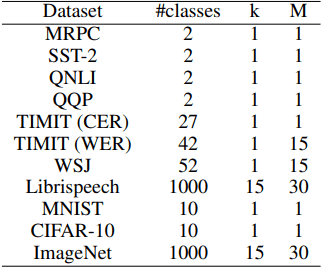## Experimental results in NLP

A comparison of cross-entropy loss and squared loss in the natural language processing task is shown below. The upper figure shows the accuracy (ACCURACY) and the lower figure shows the F1 score.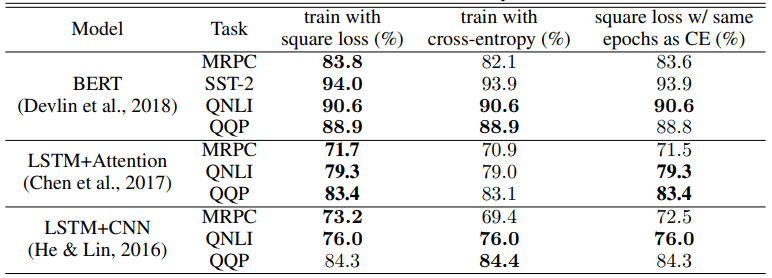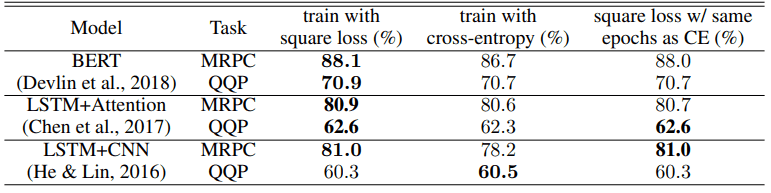In terms of accuracy, the squared loss outperforms cross-entropy for 9 out of 10 task architecture settings, and for the F1 score, the squared loss also outperforms cross-entropy learning results for 5 out of 6 settings.

Even when the number of epochs is identical (far right in the table), the squared loss shows better results for the 8/10 accuracy and 5/6 F1 score settings.

The improvement from using squared loss depends on the task model architecture, but we found that squared loss provides performance equal to or better than cross-entropy loss, except in the case of LSTM+CNN, especially in the QQP task.

## Experimental results in ASR

The comparison results for the speech recognition task are as follows.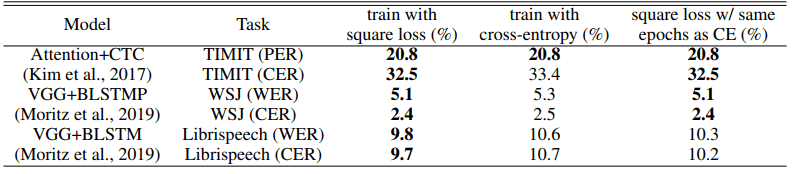PER denotes the phone error rate, CER the character error rate, and WER the word error rate (the smaller the number, the better). Under all task and model architecture settings, the squared loss is comparable to or better than the cross-entropy loss.

The relative performance difference is highest for Librispeech, which has the largest amount of data among the datasets used (9.3% for CER and 7.5% for WER).

For the next largest dataset, WSJ, the improvement in performance is about 4%, and the larger the dataset size, the higher the relative performance when using squared loss. It is not clear if this is a coincidence or if there is a property that the larger the dataset size, the better the squared loss. The number of epochs required for training was the same for TIMIT and WSJ, while Librispeech required more for squared loss, but showed better performance.

## Experimental results in CV

The results in the computer vision task are as follows.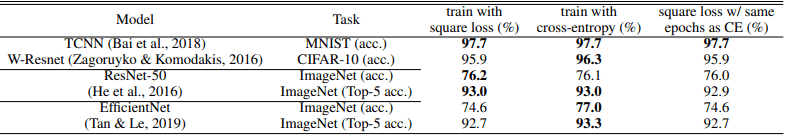Overall, the squared loss often shows the same or lower performance than when using cross-entropy loss. In particular, we can see that the performance decreases when EfficientNet is used. In addition, the number of epochs until the training converges is almost the same for all three datasets.

Compared to NLP and ASR, it can be said that the squared loss in CV did not show many advantages.

### Performance for different initializations

In order to evaluate the robustness of the model initialization to randomness, we compare the case of initialization with different random seeds. In the following figure, the difference in accuracy (or error rate) between using squared loss and using cross-entropy loss is shown.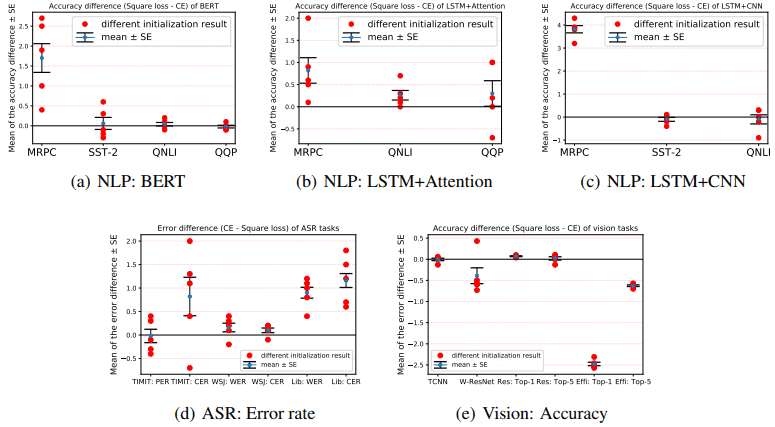The mean is indicated by a blue dot, the location of the mean ± standard deviation by a horizontal bar, and the trial results by a red dot.

The results of the standard deviation comparison are shown in the table below.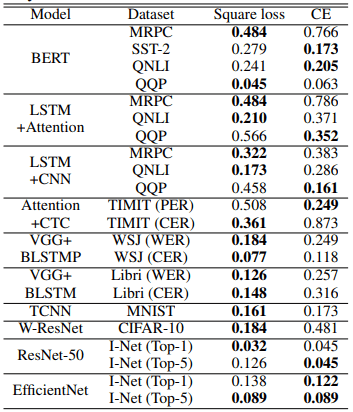Out of the 20 settings, the variance is smaller with squared loss in 15 settings. This indicates that the case using squared loss is relatively less affected by the randomness of the initialization.

### Observation of learning curve

We compare the speed of convergence of the training when the number of classes is small and large.

#### Convergence speed when the number of classes is small

The following figure shows the learning curve on the QNLI dataset. This is a two-class classification task and corresponds to a small number of classes.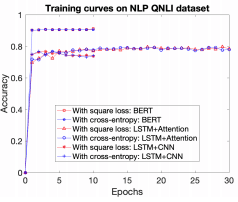All cases with squared loss are shown in red, and all cases with cross-entropy loss are shown in blue. As you can see, the convergence speed is almost the same in both cases (the accuracy is better overall with the squared loss).

#### Convergence speed when the number of classes is large

Next, as an examination of the case with a large number of classes, the learning curves for the audio dataset Librespeech and the visual dataset ImageNet are shown below.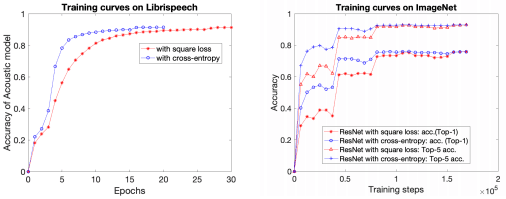As before, the red color indicates when using squared loss, and the blue color indicates when using cross-entropy loss. In Librispeech, the final performance is better when using squared loss, but the convergence speed is lower. ImageNet also shows a slight decrease in convergence speed, but the final performance is almost the same.

The convergence speed may vary depending on the nature of the task, such as the number of classes of tasks to be solved.

## summary

It is common to use cross-entropy loss in classification tasks. However, the validation results in this paper show that the squared loss is as good as or better than the cross-entropy loss in terms of accuracy, error rate, and robustness against random initialization in various tasks such as NLP, ASR, and CV. Although these results are empirical, they indicate that mean squared error in the classification task may be as effective or more effective than cross-entropy loss.

However, it should be noted that there are some results that are inferior to cross-entropy loss in terms of performance in CV tasks and convergence speed when the number of classes is large, so it is not possible to say which is always better.

Instead of using only the cross-entropy loss as the loss function in the classification task, we may want to consider the squared loss as an option.

If you have any suggestions for improvement of the content of the article,